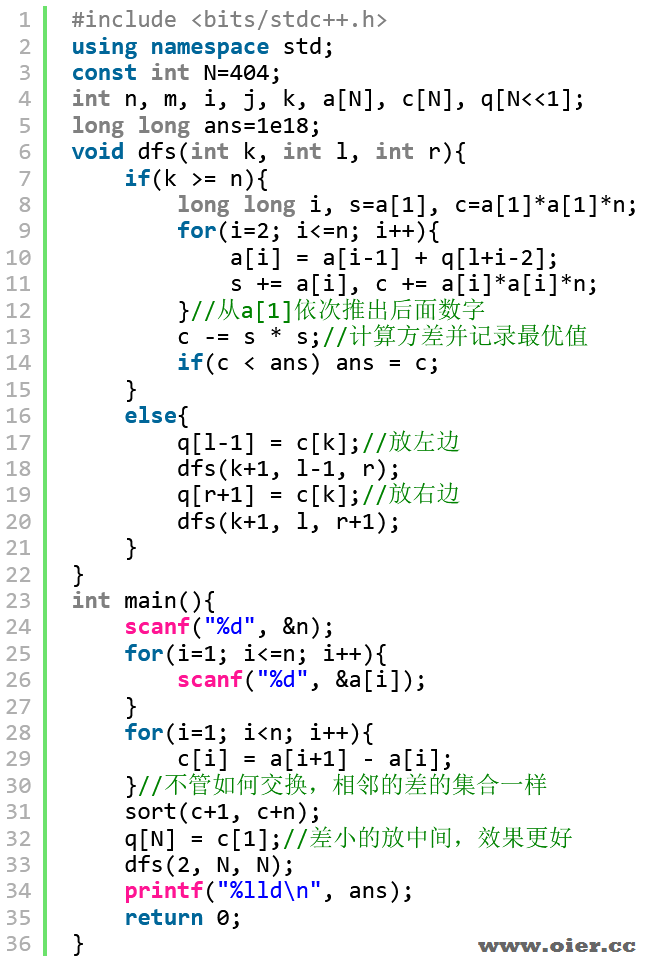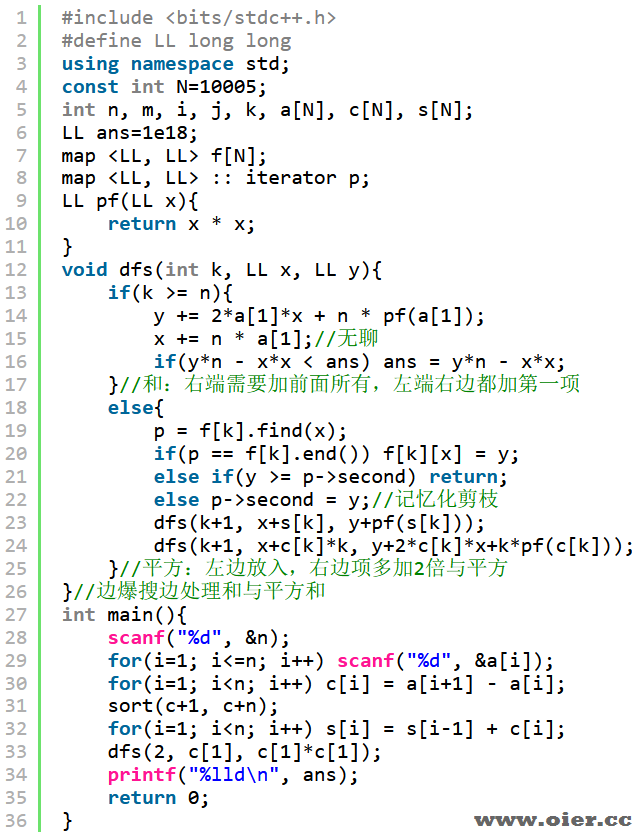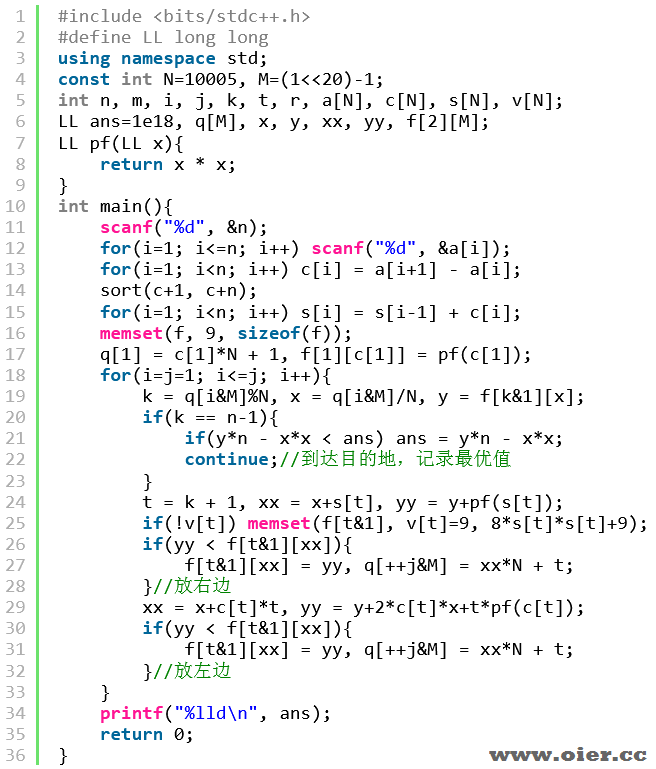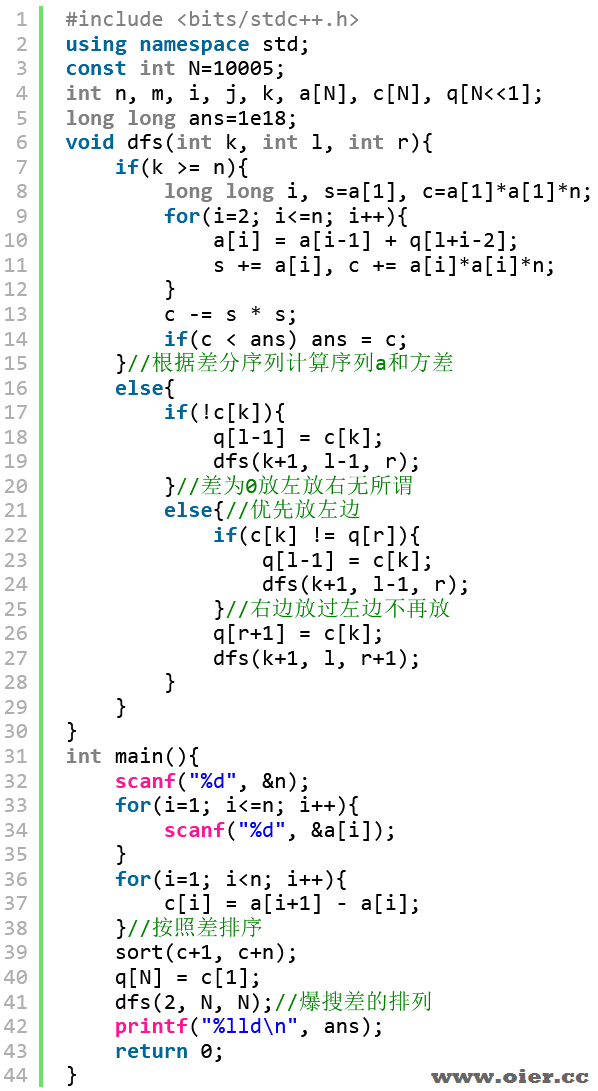392+

## 输入输出样例

### 输入样例 #1

4
1 2 4 6


### 输出样例 #1

52


### 输入样例 #2

见附件中的 variance/variance2.in

### 输出样例 #2

见附件中的 variance/variance2.ans

### 输入样例 #3

见附件中的 variance/variance3.in

### 输出样例 #3

见附件中的 variance/variance3.ans

### 输入样例 #4

见附件中的 variance/variance4.in

### 输出样例 #4

见附件中的 variance/variance4.ans

## 说明

**【样例解释 #1】**

**【数据范围】**

| 测试点编号 | $n \le$ | $a_i \le$ |
|:-:|:-:|:-:|
| $1 \sim 3$ | $4$ | $10$ |
| $4 \sim 5$ | $10$ | $40$ |
| $6 \sim 8$ | $15$ | $20$ |
| $9 \sim 12$ | $20$ | $300$ |
| $13 \sim 15$ | $50$ | $70$ |
| $16 \sim 18$ | $100$ | $40$ |
| $19 \sim 22$ | $400$ | $600$ |
| $23 \sim 25$ | ${10}^4$ | $50$ |

## 解题思路

n=4，打表找规律。

1 6 7 9、1 6 8 9、1 3 8 9、1 3 4 9、1 2 4 9、1 2 7 9……

$n*\sum{(a_i – a)^2} = n*c – 2*n*s*a + n*n*\frac{s}{n}^2 = n*c – s*s$

## 程序实现

（感谢zhangtingxi提供思路）（官方数据满分！！！）=====================爆搜AC代码====================